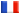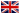﻿ Séminaire de Cryptographie - 13 janvier 2017 - Vinod Vaikuntanathan

# Séminaire de Cryptographie

## Vinod Vaikuntanathan### Low-Complexity Cryptographic Hash Functions

Cryptographic hash functions are efficiently computable functions that shrink a long input into a shorter output while achieving some of the useful security properties of a random function. The most common type of such hash functions is collision resistant hash functions (CRH), which prevent an efficient attacker from finding a pair of inputs on which the function has the same output. Despite the ubiquitous role of hash functions in cryptography, several of the most basic questions regarding their computational and algebraic complexity remained open. In this work we settle most of these questions under new, but arguably quite conservative, cryptographic assumptions, whose study may be of independent interest. Concretely, we obtain the following results: * Low-complexity CRH. Assuming the intractability of finding short codewords in natural families of linear error-correcting codes, there are CRH that shrink the input by a constant factor and have a constant algebraic degree over Z_2 (as low as 3), or even constant output locality and input locality and thus computable by linear-size circuits. Such CRH are potentially MPC- and FHE-friendly. * Win-win results. If low-degree CRH with good shrinkage do not exist, this has useful consequences for learning algorithms and data structures. * Degree-2 hash functions. Assuming the conjectured intractability of solving a random system of quadratic equations over Z_2, a uniformly random degree-2 mapping is a universal one-way hash function (UOWHF). UOWHF relaxes CRH by forcing the attacker to find a collision with a random input picked by a challenger. On the other hand, a uniformly random degree-2 mapping is not a CRH. We leave the existence of degree-2 CRH open, and relate it to open questions on the existence of degree-2 randomized encodings of functions. An important research direction is to understand the security of our assumptions from the cryptanalysis standpoint. Joint Work with Benny Applebaum, Naama Haramaty, Yuval Ishai and Eyal Kushilevitz, to appear in ITCS 2017.